# Methods for Fit Mixed Effects Model

Select the method or formula of your choice.

## The mixed effects model and the log-likelihood

### The general form of the mixed effects model

Mixed effects models contain both fixed and random effects. The general form of the mixed effects model is:

y =+ Z1μ1 + Z2μ2 + ... + Zcμc + ε

### Notation

TermDescription
ythe n x 1 vector of response values
Xthe n x p design matrix for the fixed effect terms, pn
βa p x 1 vector of unknown parameters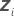the n x mi design matrix for therandom term in the model
μian mi x 1 vector of independent variables from N(0,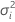)
εan n x 1 vector of independent variables from N(0,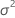)
nthe number of observations
pthe number of parameters in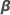cthe number of random terms in the model

### Variance-covariance matrix

Based on the model assumption for the general form of the mixed effects model, the response vector, y, has a multivariate normal distribution with mean vector and the following variance-covariance matrix:

V(σ2) = V(σ2, σ21, ... , σ2c) = σ2In + σ21Z1Z'1 + ... + σ2cZcZ'c

where

σ2 = (σ2, σ21, ... , σ2c)'

σ2, σ21, ... , σ2c are called variance components.

By factoring from the variance, you can find a representation of H(θ), which is in the computation of the log-likelihood of mixed effects models.

V(σ2) = σ2H(θ) = σ2[In + θ1Z1Z'1 + ... + θcZcZ'c]

### Notation

TermDescription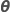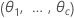θi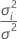, the ratio of the variance of therandom term over the error variance

### Log-likelihood

When the model contains a random factor, by default the unknown parameter estimates come from minimizing twice the negative of the restricted log-likelihood function. The minimization is equivalent to maximizing the restricted log-likelihood function. Minitab uses an iterative algorithm to minimize the restricted log-likelihood function. The function to minimize is:

### Notation

TermDescription
HIn + θ1Z1Z'1 + ... + θcZcZ'c
|H|the determinant of H
H-1the inverse of H
mithe number of levels for therandom termthe error variance component
Inthe identity matrix with n rows and columns

## Restricted Maximum Likelihood (REML) estimation

By default, Minitab calculates parameter estimates that maximize the restricted likelihood function, which is equivalent to minimizing the following function:
To minimize the function, Minitab differentiates the function with respect to β, σ2, and θi and sets the differentials equal to 0:

where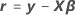Algebraic rearrangement of the first two equations to solve for the estimated parameters with respect to the differentiation give the following equations:
The derivative with respect to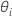cannot be explicitly solved for the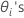. Minitab uses Newton's method to estimatewith the following steps:
1. Use the Minimum Norm Quadratic Unbiased Estimates (MINQUE)12 of the variance components to construct the initial values of σ2 and θi.
2. Estimate β and σ2 with the equations for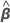and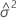.
3. Find θi with Newton's method to minimize L(β, σ2, θ).
4. Repeat steps 2 and 3 until convergence.
The converged solutions forare the variance ratio estimates. The variance component for therandom term is as follows:

### Notation

TermDescription
tr(·)the trace of the matrix
X'the transpose of X
1 Rao, C.R. (1971 a). Estimation of variance covariance components - MINQUE theory. Journal of Multivariate Analysis 1, 257–275.
2 Rao, C.R. (1971 b). Minimum variance quadratic unbiased estimation of variance components. Journal of Multivariate Analysis 1, 445–456.